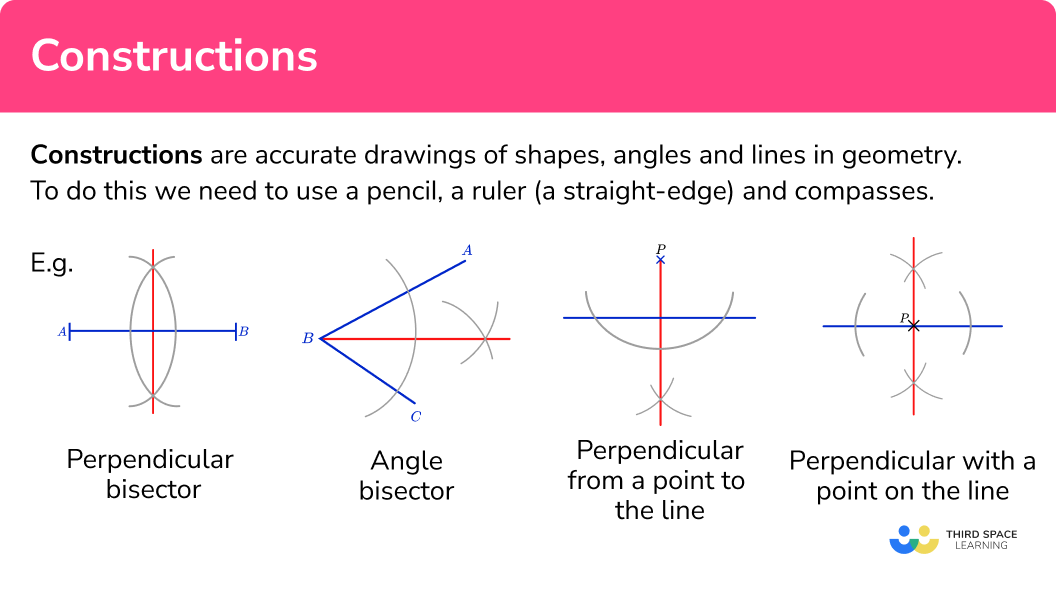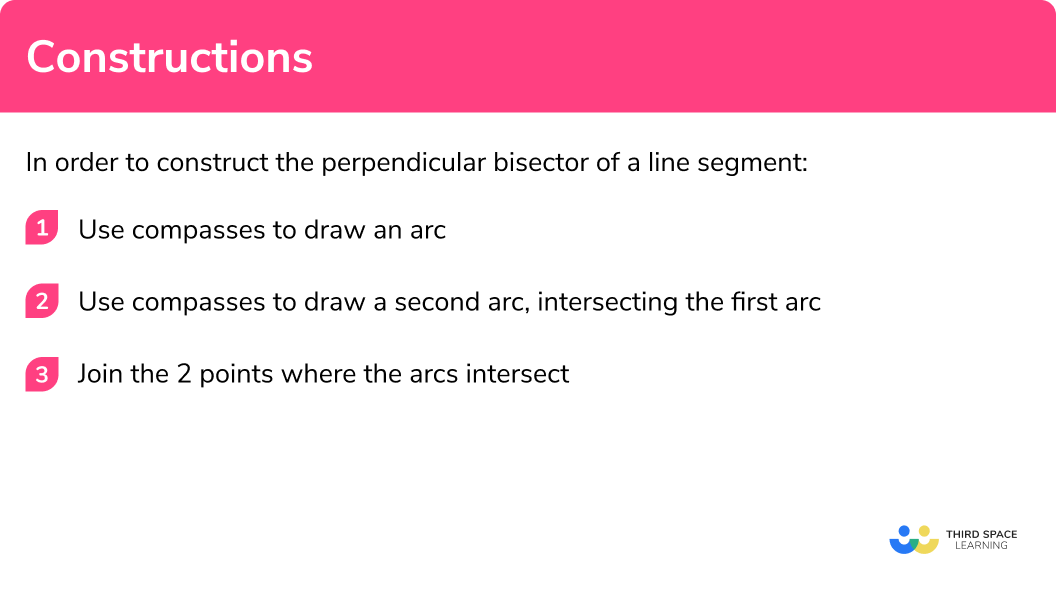# Constructions

Here we will learn about constructions, including how to draw accurately using a pencil, a ruler and a pair of compasses.

There are also constructions worksheets based on Edexcel, AQA and OCR exam questions, along with further guidance on where to go next if you’re still stuck.

## What are constructions?

Constructions are accurate drawings of shapes, angles and lines in geometry.

To do this we need to use a pencil, a ruler (a straight-edge) and compasses.

The basic constructions are perpendicular bisector and angle bisector.

In an exam you may have to construct and interpret an angle bisector, a line bisector or distance from a point.

Perpendicular bisectorAngle bisectorStep-by step-guide: Perpendicular bisector

Step-by-step guide: Angle bisector

Perpendiculars can also be constructed for a point and a given line.

Perpendicular from a point to the linePerpendicular with a point on
the lineStep-by-step guide: Constructions between points and lines

Other geometric constructions include how to draw a regular hexagon.

Step-by-step guide: How to draw a hexagon

The compass constructions can be applied on the same diagram. For example a 60 degree angle can be constructed and used to construct a 30 degree angle.

Similarly a 90 degree angle can be constructed and used to construct a 45 degree angle.

Step-by-step guide: How to construct a 30, 60, 45, 90 degree angle

### What are constructions?## Constructing regions

A locus (loci is plural) is a collection of points that have the same properties. We can use them to accurately construct lines and shapes. We can solve problems that involve intersecting loci, which require use to locate a region. To construct regions there are four ruler and compass constructions we must know:

·       Locus of all points around a point or a line.

·       Perpendicular Bisector

·       Perpendicular Bisector from a point

·       Angle Bisector

E.g.

A hospital is going to be built.

It must be no more than 10km from Town A and no more than 8km from Town B.

1cm represents 2km.

Shade the region on the diagram where the stadium can be built.

Solution:

Step 1 – draw the locus of points 10km from Town A.

Step 2 – draw the locus of points 8km from Town B.

Step 3 – shade the region where the two circles intersect. These are the points that are no more than 10km from Town A and no more than 8km from Town B.

E.g.

Here is a scale drawing of a garden.

The scale is 1cm to 2m.

A water feature is going to be placed in the garden.

The feature must be closer to AB than BC.

The feature must be less than 14m from D.

Shade the region where the water feature can be placed.

Solution:

Step 1 – construct an angle bisector to show the locus of points equidistant from AB and BC.

Step 2 – draw the locus of points 14m from point D.

Step 3 – shade the region that shows the points that are closer to Ab than DC and less than 14m from point D.

## How to construct a perpendicular bisector

In order to construct the perpendicular bisector of a line segment:

1. Use compasses to draw an arc.
2. Use compasses to draw a second arc, intersecting the first arc.
3. Join the 2 points where the arcs intersect.

### Explain how to construct a perpendicular bisector## Constructions examples

### Example 1: perpendicular bisector

Construct a perpendicular bisector of the given line AB:

1. Use compasses to draw an arc.

Open the compasses to about three-quarters of the length of the line.

Put the point of the compasses on one of the endpoints of the line.

Draw an arc.

2Use compasses to draw a second arc, intersecting the first arc.

Keeping the compasses the same, draw another arc from the other end of the line.

3Join the two points where the arcs intersect.

Using a straight-edge (a ruler), join up the two points where the arcs intersect each other.

The new line is the perpendicular bisector of the original line segment AB.

You can check that the new line goes through the midpoint of the line segment AB by using a ruler to measure. The line AB should have been cut into two equal halves at a right angle.

### Example 2: angle bisector

Construct an angle bisector of angle ABC:

Use compasses to draw an arc.

Use compasses to draw two more arcs.

Join the vertex with the point where the arcs intersect.

### Example 3: from a point to a line

Construct a line from point P perpendicular to the line.

Draw two arcs crossing the line segment.

Make two more arcs which intersect.

Join the point where the arcs intersect to the original point.

### Example 4: from a point on a line

Construct a perpendicular line passing through given point P.

Draw two arcs crossing the line segment.

Make two sets more arcs which intersect on both sides of the line.

Join the point where the arcs intersect to the original point.

### Example 5: draw a hexagon

Construct a hexagon:

Draw a circle.

Make arcs around the circle.

Join the points.

### Example 6: construct a 60 degree angle

Construct a 60^o angle:

Draw a line.

From one end of the line draw an arc.

From where the arc crosses the line, draw another arc.

Join the end point of the line to where the two arcs intersect.

### Example 7: construct a 30 degree angle

Construct a 30^o angle:

Construct a 60 degree angle.

Construct an angle bisector of the 60 degree angle.

### Example 8: construct a 90 degree angle

Construct a 90^o angle:

Draw a line.

Construct a perpendicular bisector.

### Example 9: construct a 45 degree angle

Construct a 45^o angle:

Construct a 90 degree angle .

Construct an angle bisector of the 90 degree angle.

### Example 10: all three sides

Draw the triangle ABC accurately:

Draw the base.

Set compasses for the second side and draw an arc.

Set compasses for the third side and draw an arc.

Complete the triangle.

### Example 11: two angles and the included side

Draw the triangle ABC accurately:

Draw the base.

At one end point measure one angle.

At the other end point measure the second angle.

Complete the triangle.

### Example 12: two sides and the included angle

Draw the triangle ABC accurately:

Draw the base.

At one end point measure one angle.

At the same endpoint draw an arc.

Complete the triangle.

### Common misconceptions

• The construction arcs must not be removed

The arcs drawn should be drawn lightly so can be adjusted if needed but they must be visible in your final answer. This is to show that you have used the correct method to draw the perpendicular bisector accurately.

• The pencil should be sharp

A sharp pencil helps your diagram to be accurate. Using a small pencil in compasses can also be helpful.

### Practice constructions questions

1. Construct a perpendicular bisector of the line MN.The construction must be made with compasses kept to the same setting for both arcs. The construction arcs must be seen. The perpendicular should be drawn with a straight edge.

2. Construct an angle bisector of XYZThe construction must be made with compasses kept to the same setting for the arcs.  The construction arcs must be seen. The line should be bisecting the original angle Y.

3. Construct a perpendicular from point P on the line:The construction arcs should be made with compasses and be visible. The final line should go through the original point and be drawn with a straight-edge.

4. Construct an isosceles triangle with sides 8 cm, 6 cm and 6 cmThe longest side should be 8 cm . Compasses should be used to make the arcs, and they should be clearly seen. The other two sides are the same length; 6 cm.

5. Construct a triangle ABC, with AB is 6 cm , angle BAC is 60^o and angle ABC is 45^o.Check the labelling of the triangle. The side AB should be 6 cm. The angle at A should be 60^o and angle at B should be 45^o.

6. Construct a triangle PQR, with PQ is 6 cm , QR is 5 cm and angle PQR is 60^o.Check the labelling of the triangle. The side AB should be 6 cm . The angle at A should be 60^o and angle at B should be 45^o.

### Constructions GCSE questions

1. Use a ruler and compasses to construct the perpendicular from point P to the line AB. You must show your construction lines.(2 marks)for the first arc(s) crossing the line centered on P.

(1)

for the perpendicular with all construction arcs.

(1)

2. In the space below, use a ruler and compasses to construct an equilateral triangle with sides 6 cm.

You must show all your construction lines.

One side of the triangle has already been drawn for you.(2 marks)for the construction arcs.

(1)

for the completed equilateral triangle.

(1)

3. The diagram shows the plan of a garden.A statue in the garden is equidistant from PS and from SR.

The statue is exactly 6 m from Q.

On the diagram, mark the position of the statue with a cross (\times).

(3 marks)for the angle bisector of PSR.

(1)

for arc of circle radius 6 cm centre Q.

(1)

for the correct point.

(1)

## Learning checklist

You have now learned how to:

• Construct a perpendicular bisector
• Construct an angle bisector
• Construct a perpendicular to a line including a point
• Construct a hexagon
• Construct triangles
• Construct a 30, 60, 45, 90 degree angle

## Still stuck?

Prepare your KS4 students for maths GCSEs success with Third Space Learning. Weekly online one to one GCSE maths revision lessons delivered by expert maths tutors.

Find out more about our GCSE maths tuition programme.